Anzeige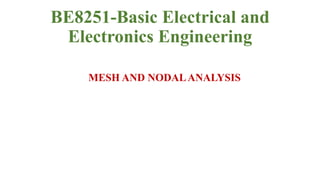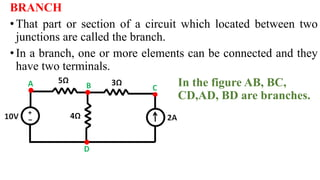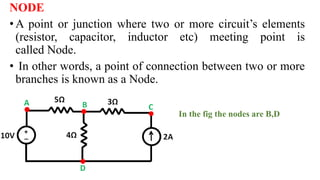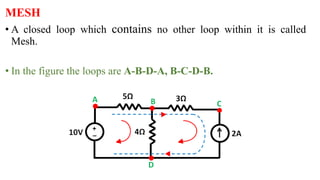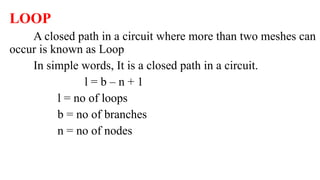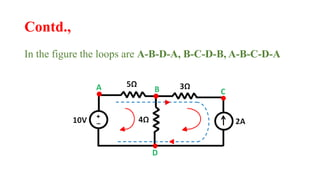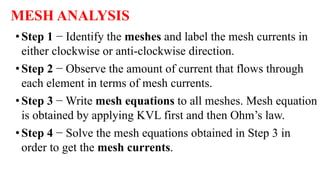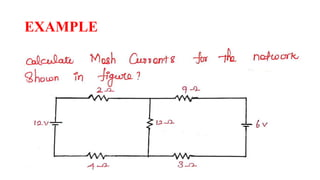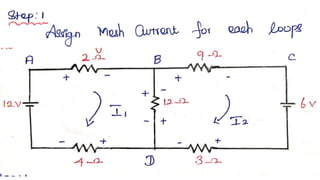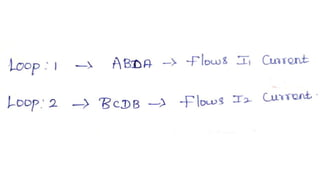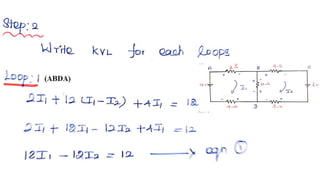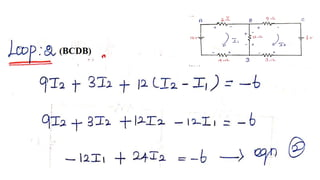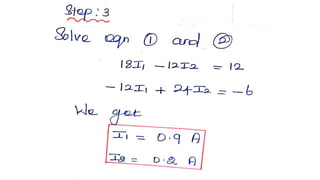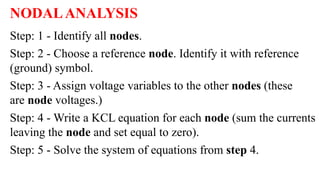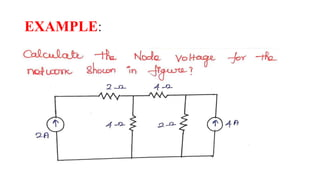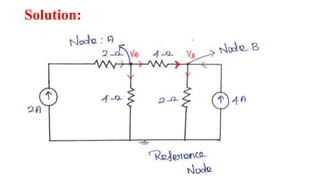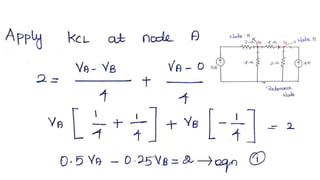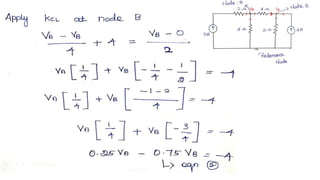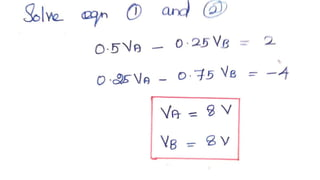1 von 20
Anzeige

### mesh node.pptx

1. BE8251-Basic Electrical and Electronics Engineering MESH AND NODALANALYSIS
2. BRANCH •That part or section of a circuit which located between two junctions are called the branch. •In a branch, one or more elements can be connected and they have two terminals. In the figure AB, BC, CD,AD, BD are branches.
3. NODE • A point or junction where two or more circuit’s elements (resistor, capacitor, inductor etc) meeting point is called Node. • In other words, a point of connection between two or more branches is known as a Node. • • In the fig the nodes are B,D
4. MESH • A closed loop which contains no other loop within it is called Mesh. • In the figure the loops are A-B-D-A, B-C-D-B.
5. LOOP A closed path in a circuit where more than two meshes can occur is known as Loop In simple words, It is a closed path in a circuit. l = b – n + 1 l = no of loops b = no of branches n = no of nodes
6. Contd., In the figure the loops are A-B-D-A, B-C-D-B, A-B-C-D-A
7. MESH ANALYSIS •Step 1 − Identify the meshes and label the mesh currents in either clockwise or anti-clockwise direction. •Step 2 − Observe the amount of current that flows through each element in terms of mesh currents. •Step 3 − Write mesh equations to all meshes. Mesh equation is obtained by applying KVL first and then Ohm’s law. •Step 4 − Solve the mesh equations obtained in Step 3 in order to get the mesh currents.
8. EXAMPLE
9. NODALANALYSIS Step: 1 - Identify all nodes. Step: 2 - Choose a reference node. Identify it with reference (ground) symbol. Step: 3 - Assign voltage variables to the other nodes (these are node voltages.) Step: 4 - Write a KCL equation for each node (sum the currents leaving the node and set equal to zero). Step: 5 - Solve the system of equations from step 4.
10. EXAMPLE:
11. Solution:
12. Thank you
Anzeige